# Rate LawsPage 2

#### WATCH ALL SLIDES

2

+

1

= 3

 The reaction is 3rd order

Slide 11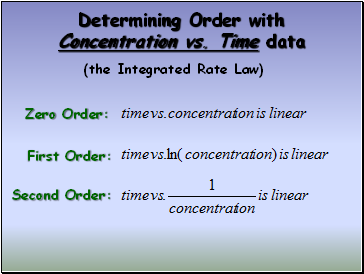## Determining Order with Concentration vs. Time data

(the Integrated Rate Law)

Zero Order:

First Order:

Second Order:

Slide 12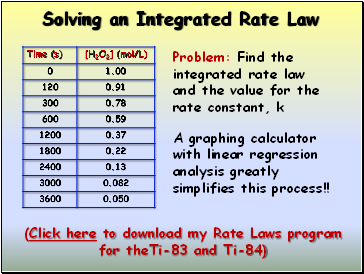## Solving an Integrated Rate Law

Problem: Find the integrated rate law and the value for the rate constant, k

A graphing calculator with linear regression analysis greatly simplifies this process!!

Slide 13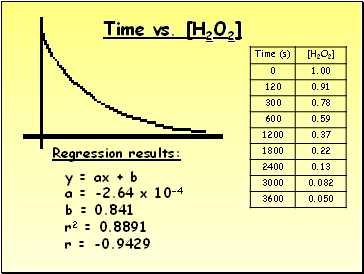Time vs. [H2O2]

y = ax + b

a = -2.64 x 10-4

b = 0.841

r2 = 0.8891

r = -0.9429

Regression results:

Slide 14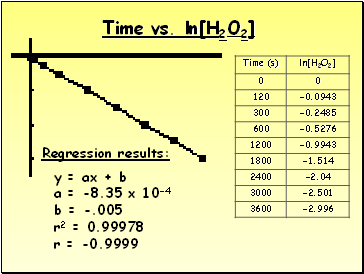Time vs. ln[H2O2]

Regression results:

y = ax + b

a = -8.35 x 10-4

b = -.005

r2 = 0.99978

r = -0.9999

Slide 15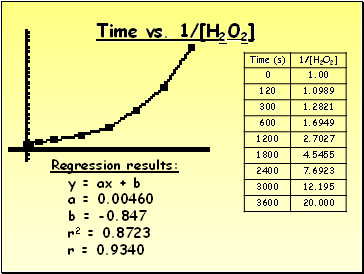Time vs. 1/[H2O2]

y = ax + b

a = 0.00460

b = -0.847

r2 = 0.8723

r = 0.9340

Regression results:

Slide 16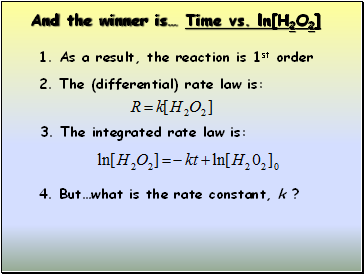And the winner is… Time vs. ln[H2O2]

1. As a result, the reaction is 1st order

2. The (differential) rate law is:

3. The integrated rate law is:

4. But…what is the rate constant, k ?

Slide 17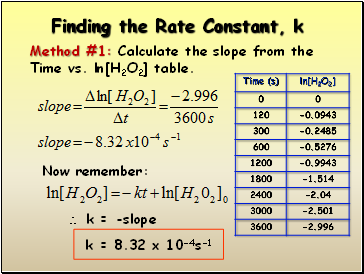## Finding the Rate Constant, k

Method #1: Calculate the slope from the

Time vs. ln[H2O2] table.

Now remember:

 k = -slope

k = 8.32 x 10-4s-1

Slide 18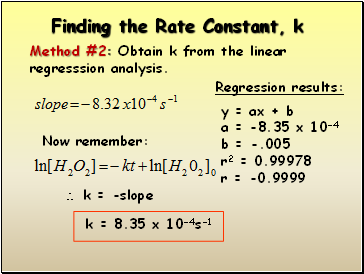Finding the Rate Constant, k

Method #2: Obtain k from the linear regresssion analysis.

Now remember:

 k = -slope

k = 8.35 x 10-4s-1

Regression results:

y = ax + b

a = -8.35 x 10-4

b = -.005

r2 = 0.99978

r = -0.9999

Slide 19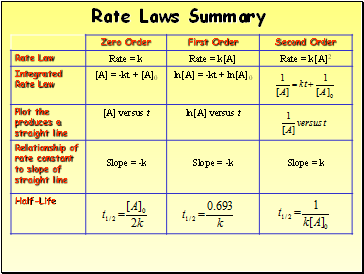Go to page:
1  2